#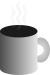Coffee Space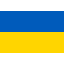Listen:

## Colour Selection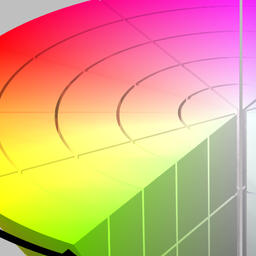Preview Image

Recently I found that I need to be able to produce near infinite colours to display on a graph as lines, like so: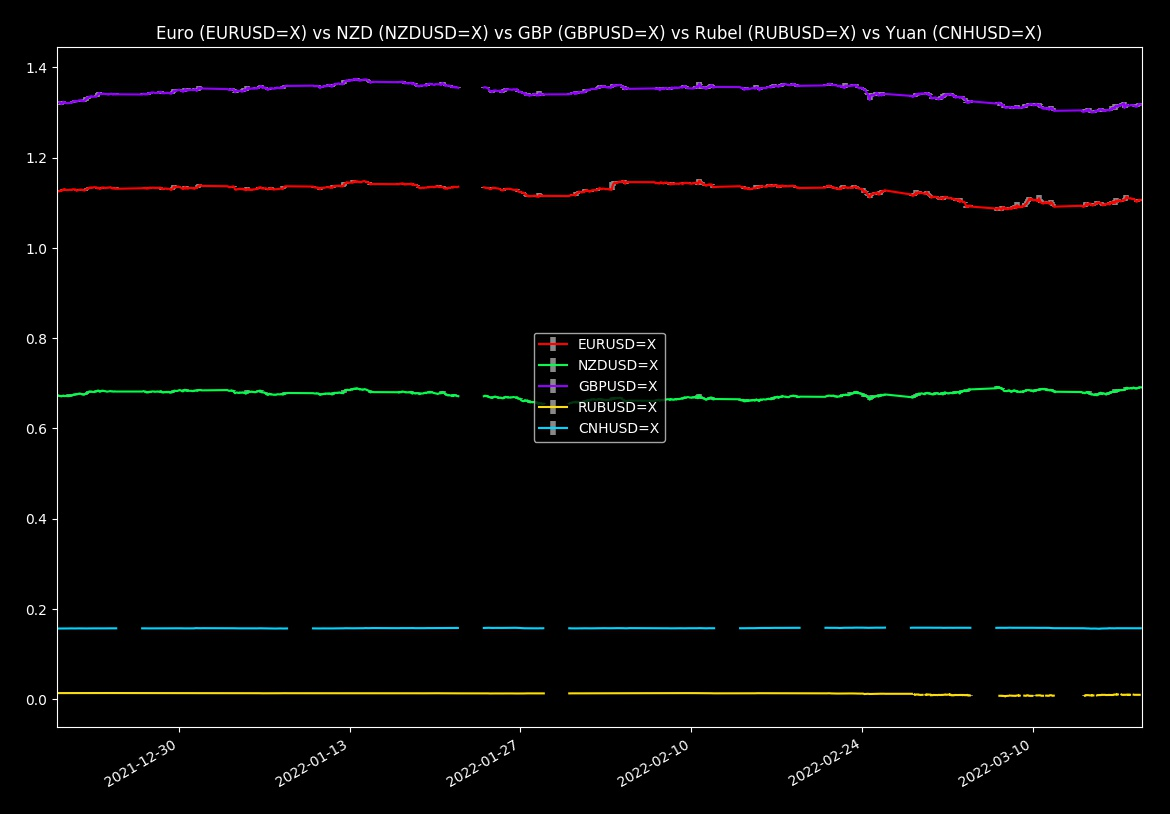Plot of currency exchange market

I have solved this problem before, but completely forgot how I did it. There isn't so much about colour selection online, so I will discuss this quickly here...

Problem definition: Given some number, $i$, where $i\ge 0$ and $i$ counts upwards such that $i=\left\{0,1,..,n\right\}$, produce a unique HTML colour that minimizes overlap with existing colours.

This article was inspired by a conversation with a friend many moons ago...

### Colour Theory

Note: This is just surface deep - colour theory gets much harder. There be dragons here!

The RGB colour space is not so easy to work with, as you have to adjust three different values to produce some colour 1.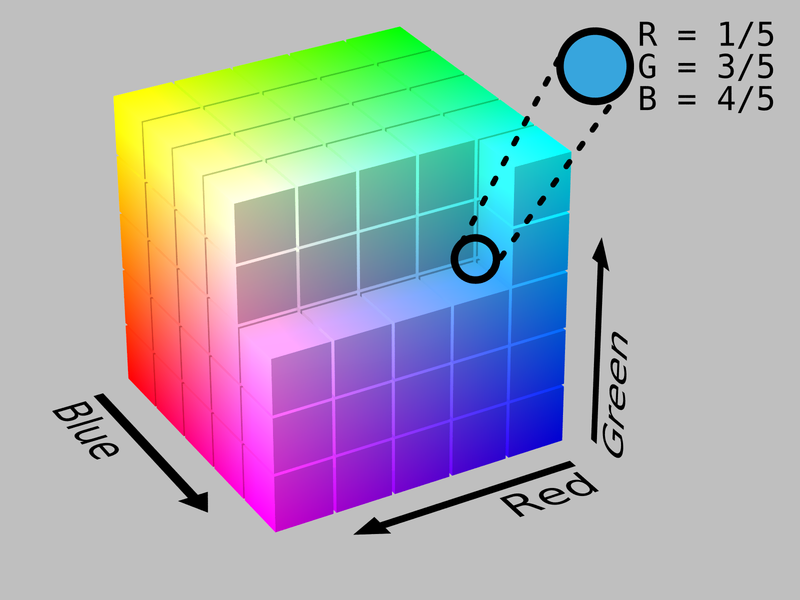RGB colour space

Instead, we look to pick a colour from the HSV colour space 2.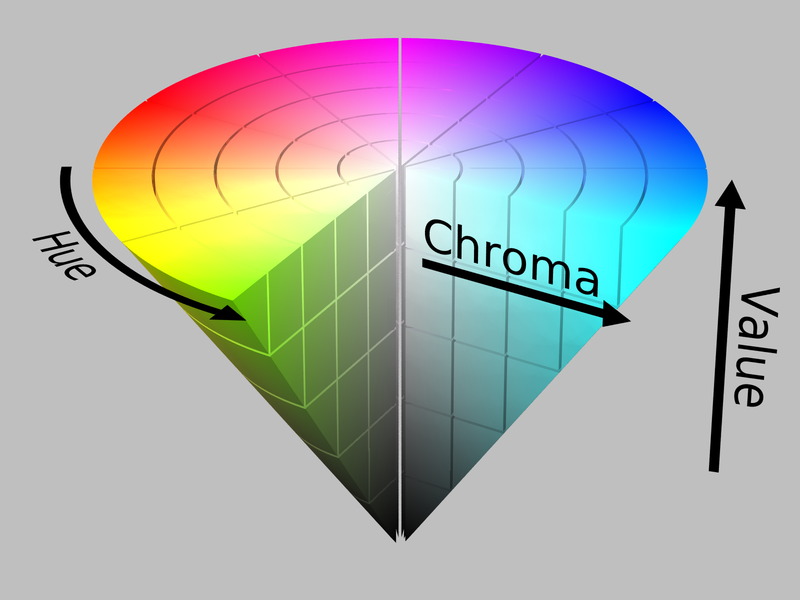RGB colour space

By changing just the hue and locking in the saturation and value, we can adjust one parameter and select distinctively different colours.

### Natural Selection

In nature flowers like to grow florets such that they maximize sunlight, by minimizing overlap 3.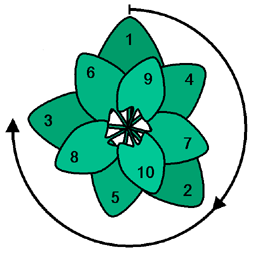Flower golden angle

You can checkout the Wikipedia article for more information on this, but we are interested in the following section:

The fraction of a circle occupied by the golden angle is therefore

$f\approx 0.381966$

### Code

Without further delay, here the is the code that solves the problem:

```0001 from matplotlib.colors import hsv_to_rgb
0002 import numpy as np
0003
0004 # get_col()
0005 #
0006 # Get a HTML RGB colour based on an index number.
0007 #
0008 # @param i The index number.
0009 # @return The suggested HTML colour.
0010 def get_col(i) :
0011   # hsv_to_rgb() function takes values between 0.0 and 1.0
0012   # Multiply the index value by golden angle number and mod it
0013   rgb = hsv_to_rgb(np.array([(i * 0.381966) % 1.0, 1, 1]))
0014   # hsv_to_rgb() returns an array of 3 values between 0.0 and 1.0
0015   # Generate RGB bytes
0016   r = int(rgb * 255)
0017   g = int(rgb * 255)
0018   b = int(rgb * 255)
0019   # Now put into a single integer representing the colour
0020   c = (r << 16) | (g << 8) | b
0021   # Convert to a hex string and remove '0x' from the front
0022   r = hex(c)[2:]
0023   # Ensure we end up with 6 characters
0024   while len(r) < 6 :
0025     r = "0" + r
0026   # Throw a '#' on the front to indicate it is a HTML colour
0027   return "#" + r```

As you can see, we borrow the `hsv_to_rgb()` conversion from `matplotlib` (as I wanted to use this library anyway) 4.

Use at your own risk. It works for me - maybe there is still some edge cases, i.e. there is no guarding for $i<0$, or any testing for large values of $i$ (it will repeat eventually). A better algorithm may also make use of darker colours, or have the ability to select which ranges you want to pick from for a 'theme'.

1. Note: You could remove this dependency by writing the conversion yourself.